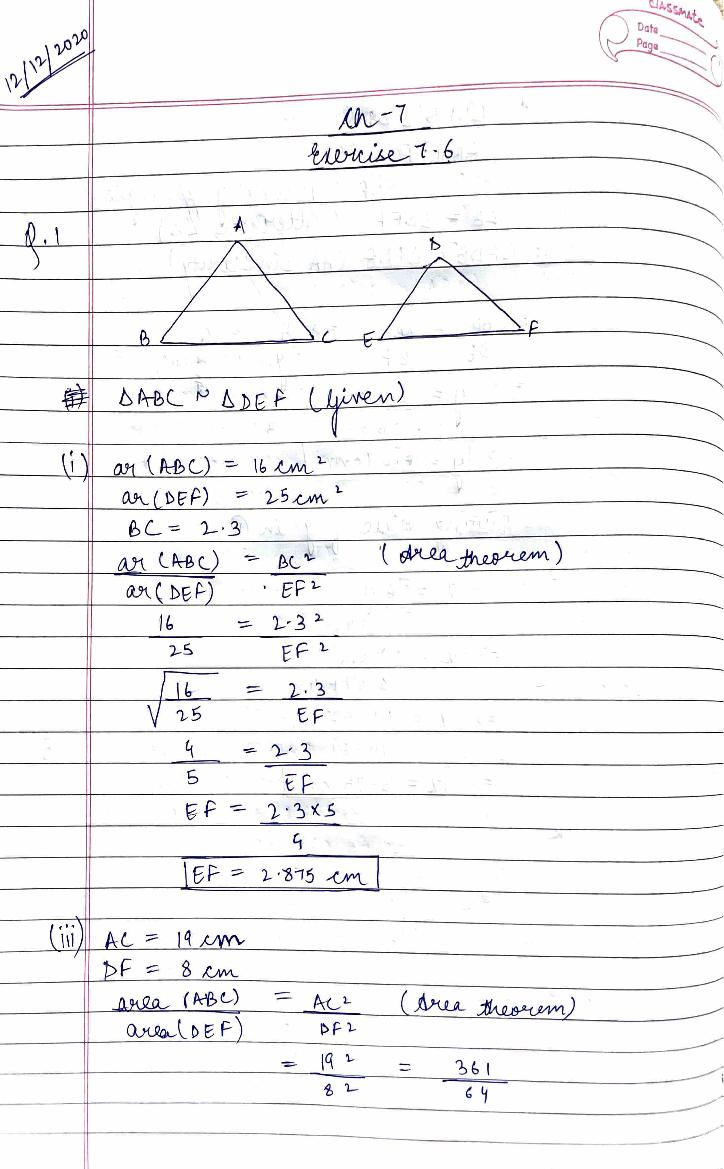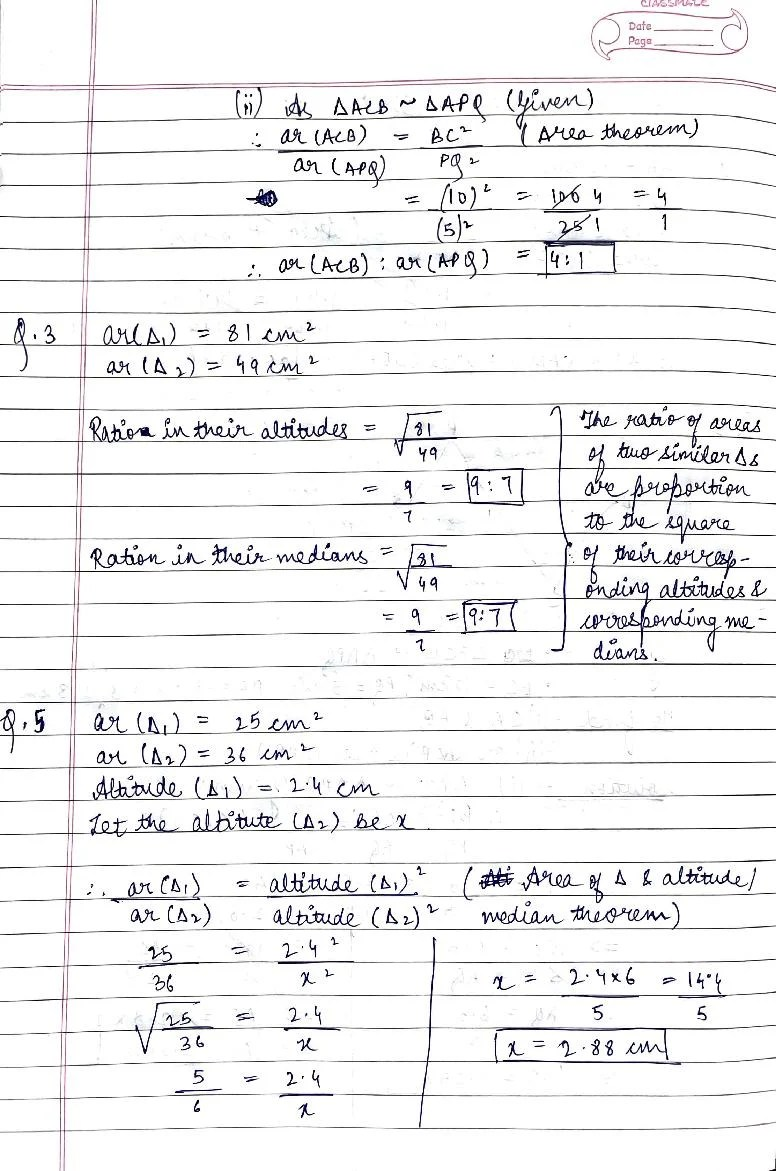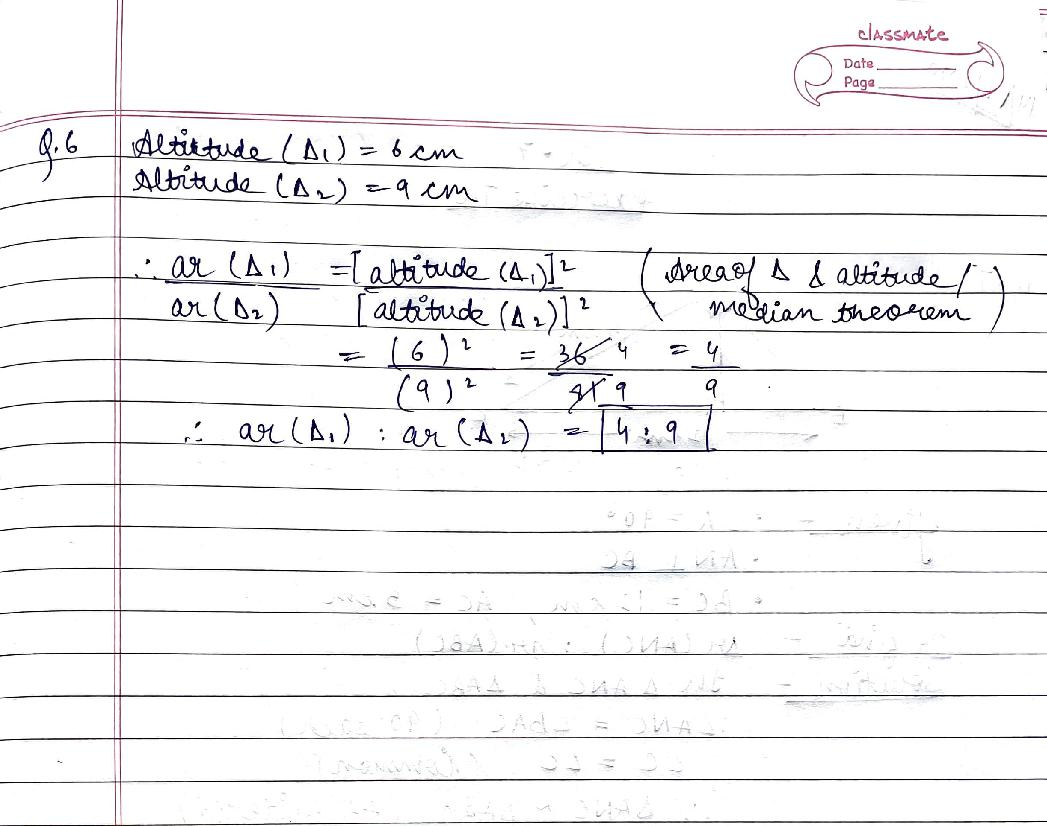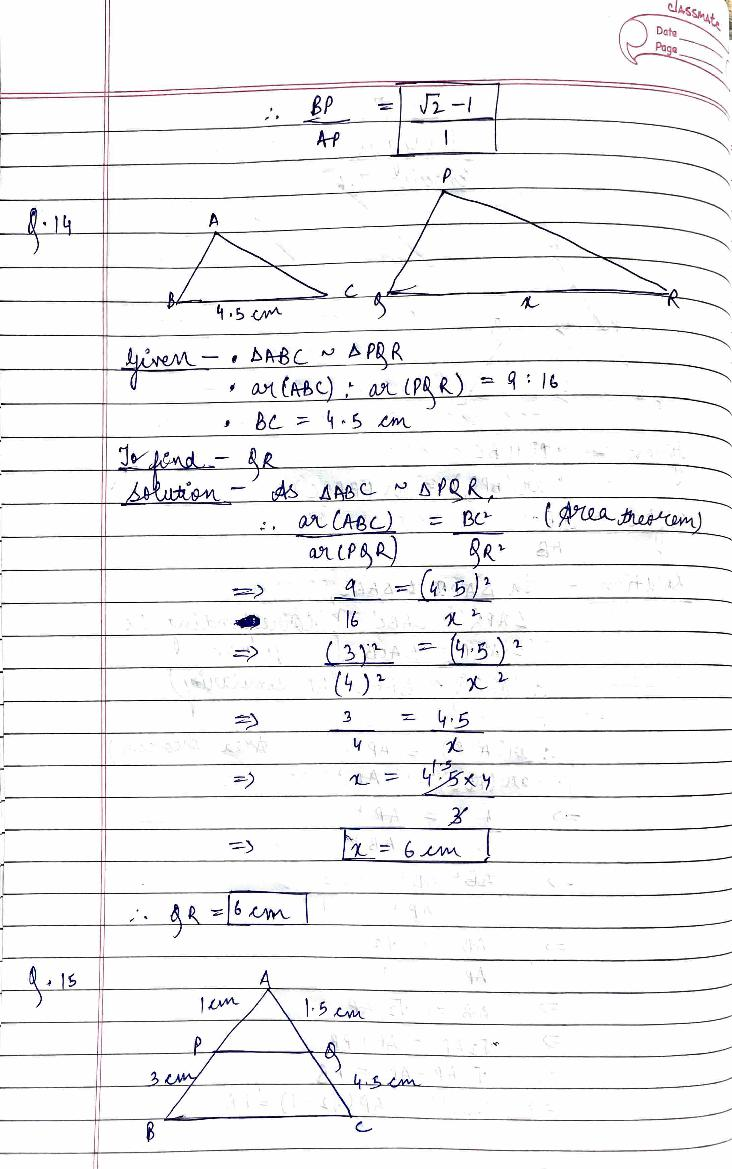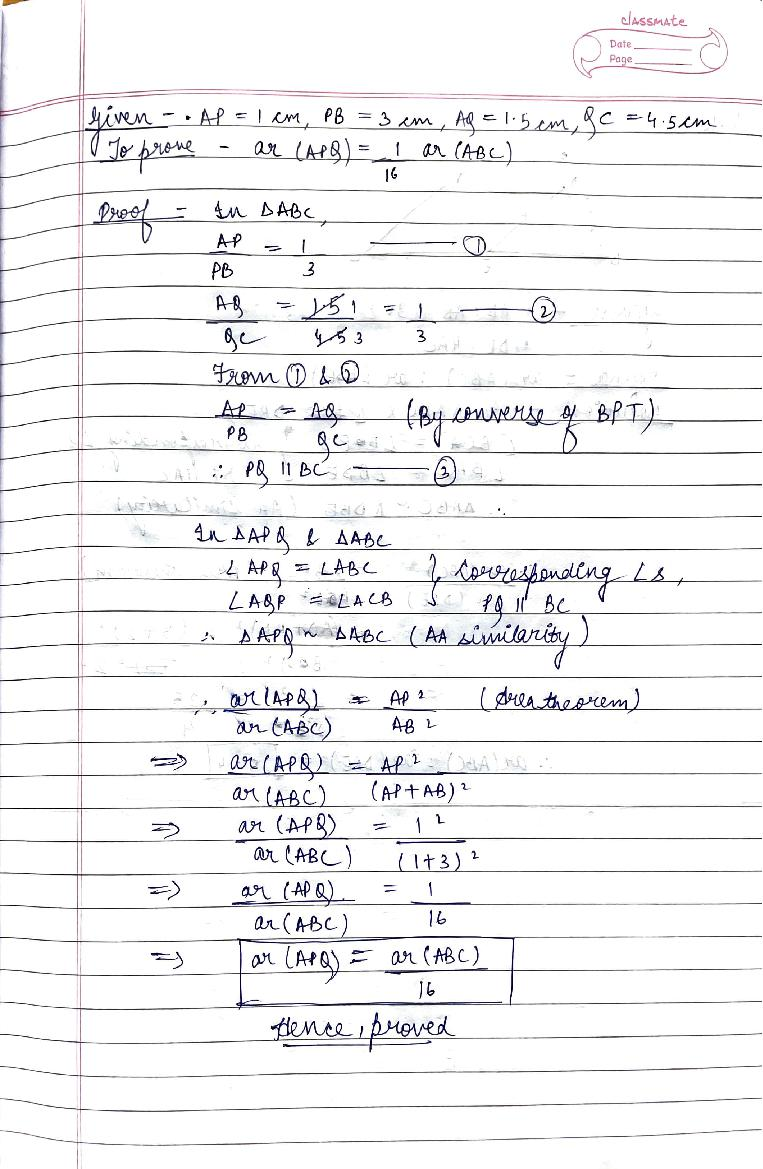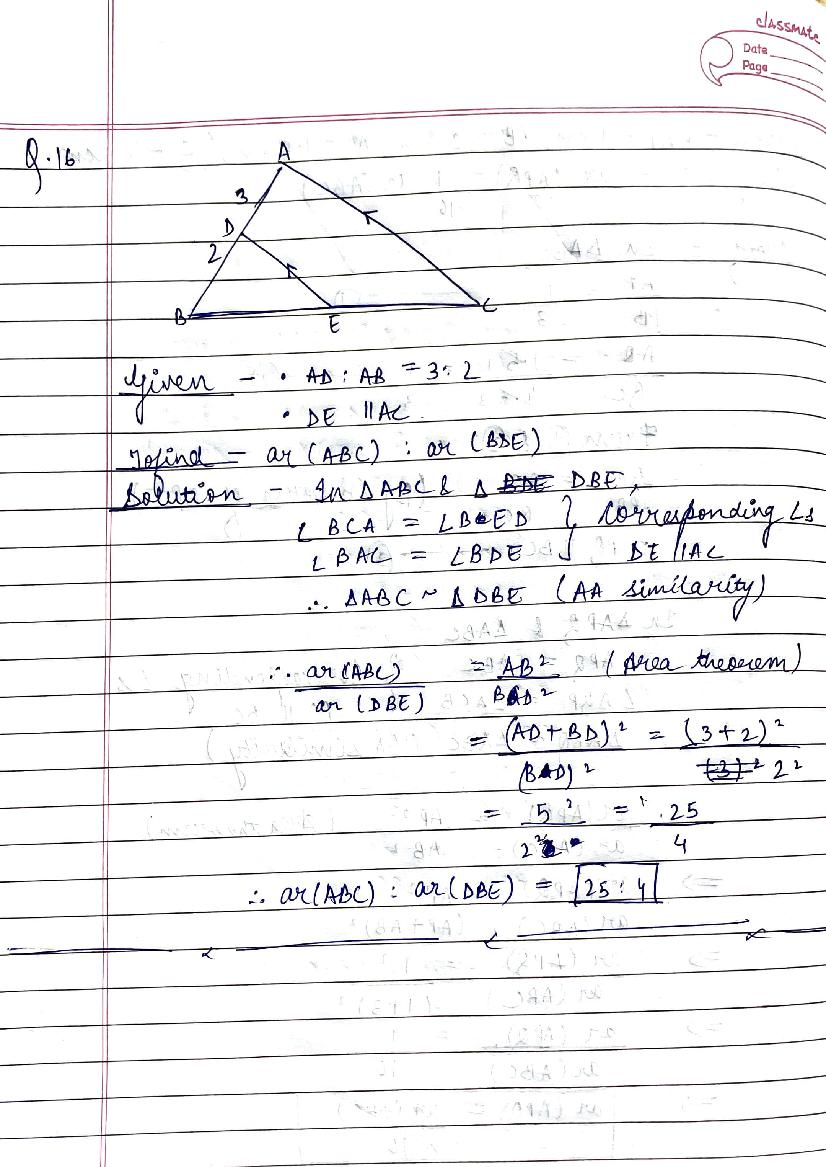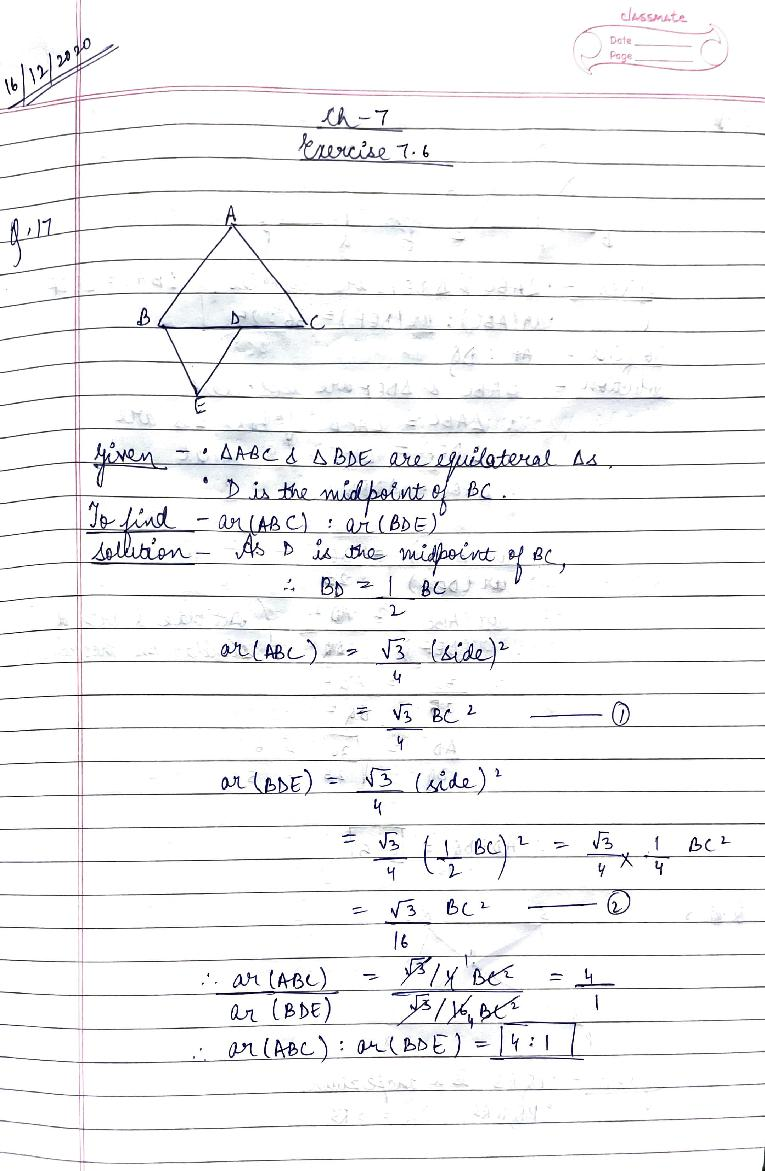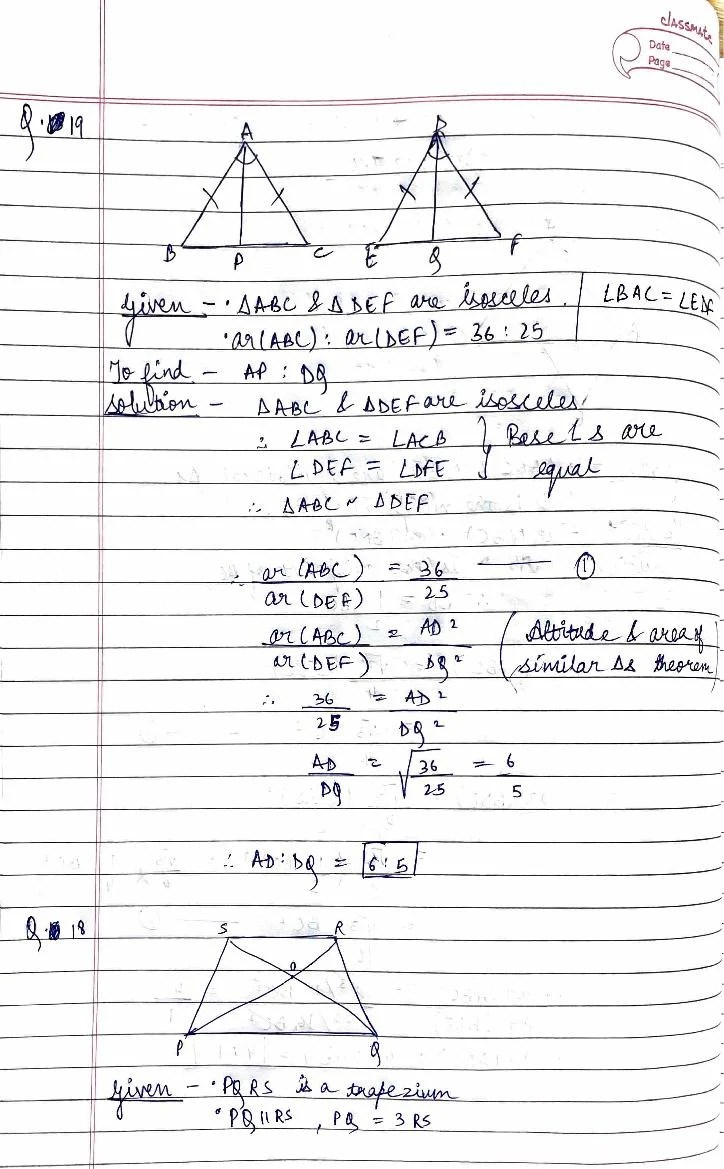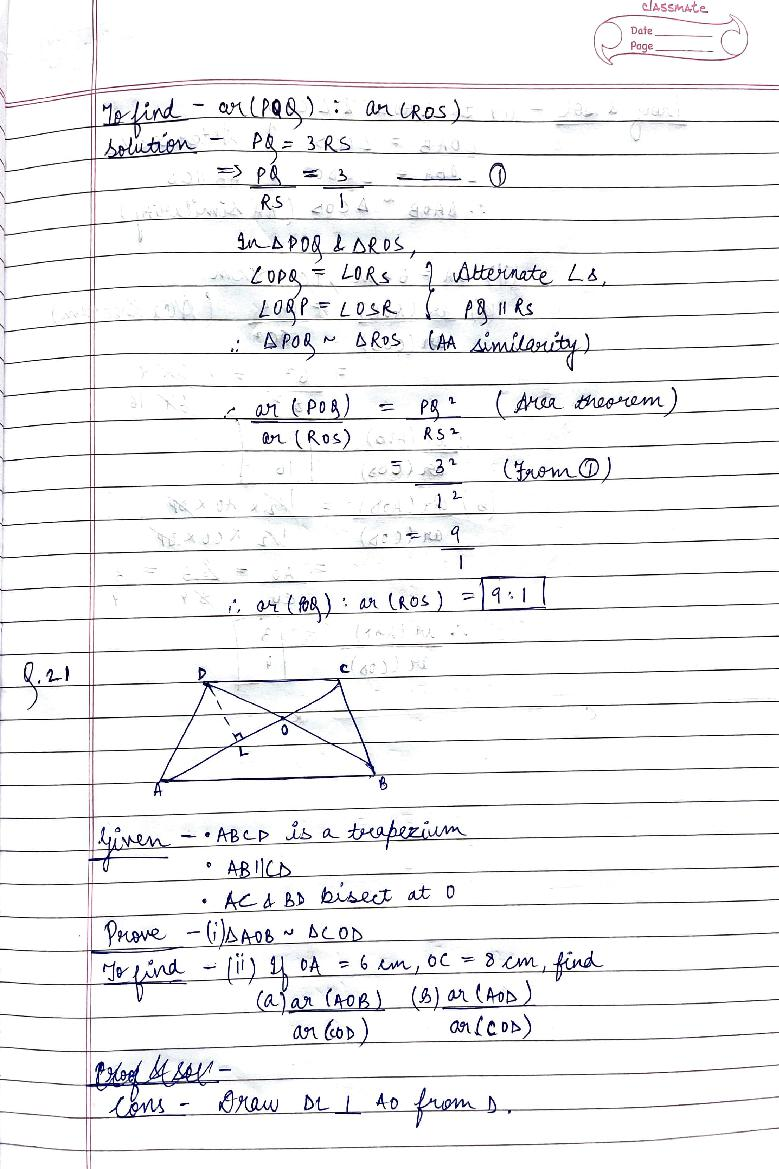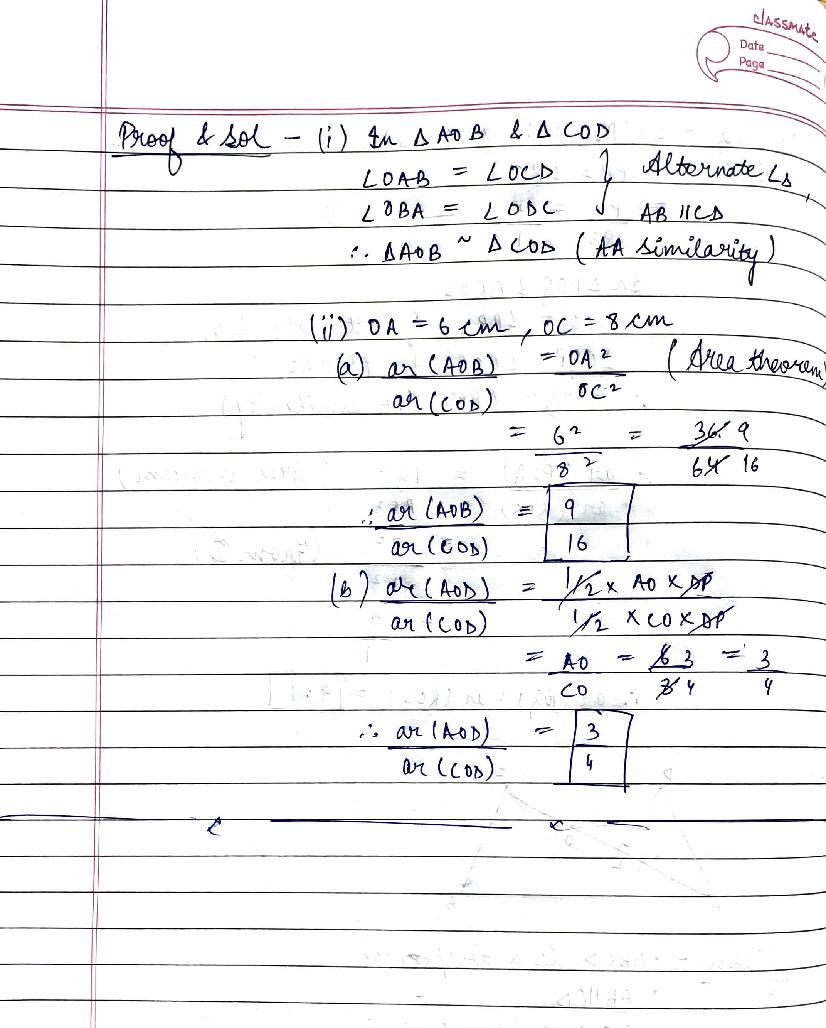# RD Sharma Solutions Class 10 Chapter 7 Triangles Exercise 7.6

Here you can get free RD Sharma Solutions for Class 10 Maths Chapter 7 Triangles Exercise 7.6. All RD Sharma Book Solutions are given here exercise wise for the chapter Triangles. RD Sharma Solutions are helpful in the preparation of several school level, graduate and undergraduate level competitive exams. Practicing questions from RD Sharma Mathematics Solutions for Class 10 Chapter 7 Triangles is proven to enhance your math skills.

 Class: Class 10th Chapter: Chapter 7 Exercise: Exercise 7.6 Name: Triangles

## RD Sharma Solutions Class 10 Chapter 7 Triangles Exercise 7.6

RD Sharma Class 10 Solutions Chapter 7 for Triangles Exercise 7.6 are given below.

RD Sharma Solutions Class 10 Chapter 7 Triangles Exercise 7.6